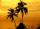Powers

Express the expressionas the n-th power of the base 10.

Result

n =  309

Solution:Leave us a comment of example and its solution (i.e. if it is still somewhat unclear...):

Showing 1 comment:Math student
Help I need help I don’t get itNext similar examples:

1. One halfOne half of ? is: ?
2. EquationHow many real roots has equation ? ?
3. PowerNumber ?. Find the value of x.
4. Eight palmThere grows 8 palms by the sea. At the first sitting one parrot, on second two, on third sits four parrots on each other twice the previous parrots sitting on a previous palm. How many parrots sitting on eighth palm?
5. 6 termsFind the first six terms of the sequence. a1 = 7, an = an-1 + 6
6. Nineteenth memberFind the nineteenth member of the arithmetic sequence: a1=33 d=5 find a19
7. TreesA certain species of tree grows an average of 0.5 cm per week. Write an equation for the sequence that represents the weekly height of this tree in centimeters if the measurements begin when the tree is 200 centimeters tall.
8. Sequence 2Write the first 5 members of an arithmetic sequence a11=-14, d=-1
9. Functions f,gFind g(1) if g(x) = 3x - x2 Find f(5) if f(x) = x + 1/2
10. Linear systemSolve a set of two equations of two unknowns: 1.5x+1.2y=0.6 0.8x-0.2y=2
11. Factory and divisionsThe factory consists of three auxiliary divisions total 2,406 employees. The second division has 76 employees less than 1st division and 3rd division has 212 employees more than the 2nd. How many employees has each division?
12. Two equationsSolve equations (use adding and subtracting of linear equations): -4x+11y=5 6x-11y=-5
13. Volleyball8 girls wants to play volleyball against boys. On the field at one time can be six players per team. How many initial teams of this girls may trainer to choose?
14. ConfectioneryThe village markets have 5 kinds of sweets, one weighs 31 grams. How many different ways a customer can buy 1.519 kg sweets.
15. The ballThe ball was discounted by 10 percent and then again by 30 percent. How many percent of the original price is now?
16. Hotel roomsIn the 45 rooms, there were 169 guests, some rooms were three-bedrooms and some five-bedrooms. How many rooms were?
17. Theorem proveWe want to prove the sentence: If the natural number n is divisible by six, then n is divisible by three. From what assumption we started?